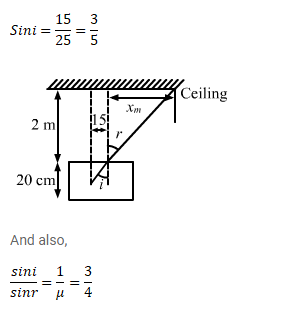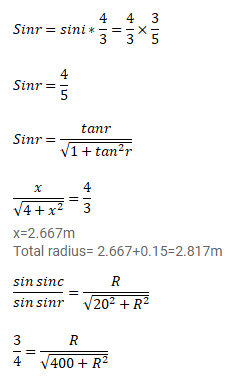# A container contains water up to a heightQuestion:

A container contains water up to a height of $20 \mathrm{~cm}$ and there is a point source at the center of the bottom of the container. A rubber ring of radius $r$ floats centrally on the water surface. (a) Find the radius of the shadow of the ring formed on the ceiling if $r=15 \mathrm{~cm}$ (b) Find the maximum value of $r$ which the shadow of the ring is formed on the ceiling refractive index of water $=4 / 3$.

Solution:$R=22.67 \mathrm{~cm}$